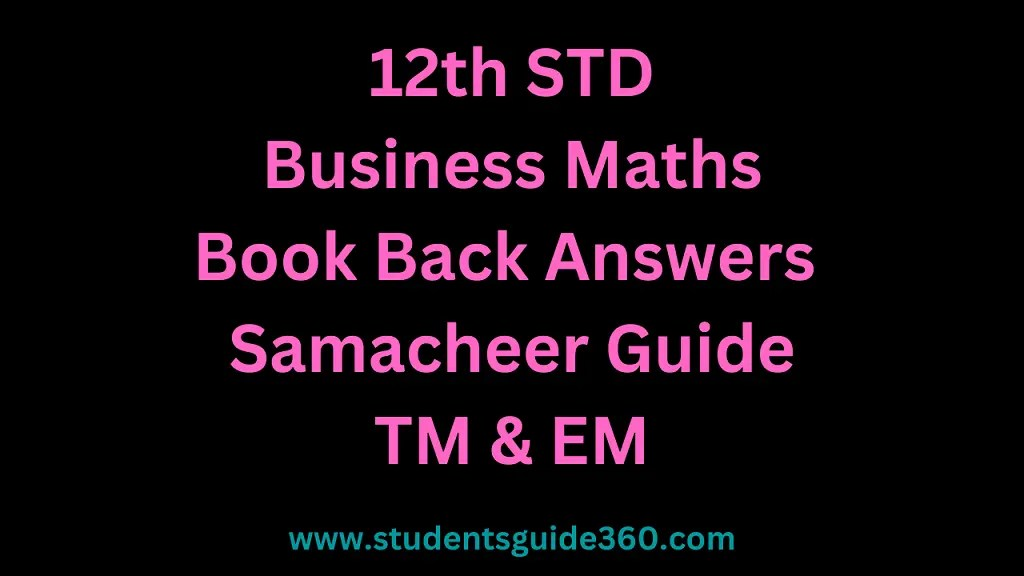12th standard Business Maths Book Back Answers. Class 12 Samacheer Kalvi 12th Business Maths Guide Book Back Answers. Class 1 to 12 Book Back Answers. Class 12th BM Chapter 1 to Chapter 18 Book Back and additional questions and answers. 12th Public Model Question Paper.## 12th Business Maths Volume 1 Solution – Book Back Answers Guide

#### 12th Business Maths Guide Chapter 1 Applications of Matrices and Determinants

• Chapter 1 Applications of Matrices and Determinants Exercise 1.1
• Chapter 1 Applications of Matrices and Determinants Exercise 1.2
• Chapter 1 Applications of Matrices and Determinants Exercise 1.3
• Chapter 1 Applications of Matrices and Determinants Exercise 1.4
• Chapter 1 Applications of Matrices and Determinants Miscellaneous Problems

#### 12th Business Maths Book Back Answers Chapter 2 Integral Calculus I

• Chapter 2 Integral Calculus I Exercise 2.1
• Chapter 2 Integral Calculus I Exercise 2.2
• Chapter 2 Integral Calculus I Exercise 2.3
• Chapter 2 Integral Calculus I Exercise 2.4
• Chapter 2 Integral Calculus I Exercise 2.5
• Chapter 2 Integral Calculus I Exercise 2.6
• Chapter 2 Integral Calculus I Exercise 2.7
• Chapter 2 Integral Calculus I Exercise 2.8
• Chapter 2 Integral Calculus I Exercise 2.9
• Chapter 2 Integral Calculus I Exercise 2.10
• Chapter 2 Integral Calculus I Exercise 2.11
• Chapter 2 Integral Calculus I Exercise 2.12
• Chapter 2 Integral Calculus I Miscellaneous Problems

#### 12th Business Maths Book Back Answers Chapter 3 Integral Calculus II

• Chapter 3 Integral Calculus II Exercise 3.1
• Chapter 3 Integral Calculus II Exercise 3.2
• Chapter 3 Integral Calculus II Exercise 3.3
• Chapter 3 Integral Calculus II Exercise 3.4
• Chapter 3 Integral Calculus II Miscellaneous Problems

#### 12th std Business Maths Guide Chapter 4 Differential Equations

• Chapter 4 Differential Equations Exercise 4.1
• Chapter 4 Differential Equations Exercise 4.2
• Chapter 4 Differential Equations Exercise 4.3
• Chapter 4 Differential Equations Exercise 4.4
• Chapter 4 Differential Equations Exercise 4.5
• Chapter 4 Differential Equations Exercise 4.6
• Chapter 4 Differential Equations Miscellaneous Problems

#### TN 12th Business Maths Guide Volume 1 Chapter 5 Numerical Methods

• Chapter 5 Numerical Methods Exercise 5.1
• Chapter 5 Numerical Methods Exercise 5.2
• Chapter 5 Numerical Methods Exercise 5.3
• Chapter 5 Numerical Methods Miscellaneous Problems

## 12th Business Maths Volume 2 Solution – Book Back Answers Guide

#### 12th Business Maths Guide Volume 2 – Random Variable and Mathematical Expectation

• Chapter 6 Random Variable and Mathematical Expectation Exercise 6.1
• Chapter 6 Random Variable and Mathematical Expectation Exercise 6.2
• Chapter 6 Random Variable and Mathematical Expectation Exercise 6.3
• Chapter 6 Random Variable and Mathematical Expectation Miscellaneous Problems

#### 12th Business Maths Guide Chapter 7 Probability Distributions

• Chapter 7 Probability Distributions Exercise 7.1
• Chapter 7 Probability Distributions Exercise 7.2
• Chapter 7 Probability Distributions Exercise 7.3
• Chapter 7 Probability Distributions Exercise 7.4
• Chapter 7 Probability Distributions Miscellaneous Problems

#### 12th Business Maths Guide Chapter 8 Sampling Techniques and Statistical Inference

• Chapter 8 Sampling Techniques and Statistical Inference Exercise 8.1
• Chapter 8 Sampling Techniques and Statistical Inference Exercise 8.2
• Chapter 8 Sampling Techniques and Statistical Inference Exercise 8.3
• Chapter 8 Sampling Techniques and Statistical Inference Miscellaneous Problems

• Chapter 9 Applied Statistics Exercise 9.1
• Chapter 9 Applied Statistics Exercise 9.2
• Chapter 9 Applied Statistics Exercise 9.3
• Chapter 9 Applied Statistics Exercise 9.4
• Chapter 9 Applied Statistics Miscellaneous Problems

#### 12th Business Maths solution Chapter 10 Operations Research

• Chapter 10 Operations Research Exercise 10.1
• Chapter 10 Operations Research Exercise 10.2
• Chapter 10 Operations Research Exercise 10.3
• Chapter 10 Operations Research Exercise 10.4
• Chapter 10 Operations Research Miscellaneous Problems